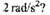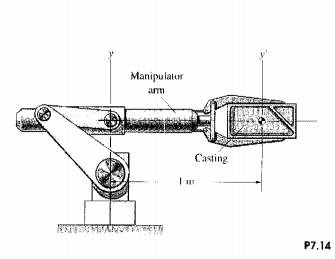### Create an Account

Home / Questions / The mass moment of inertia of the robotic manipulator arm about the vertical y axis is 10 ...

# The mass moment of inertia of the robotic manipulator arm about the vertical y axis is 10 kg m2 The mass moment of inertia of the 14kg casting held by the arm about the y' axis is 08kgm2 What

The mass moment of inertia of the robotic manipulator arm about the vertical y axis is 10 kg. m2 " The mass moment of inertia of the 14kg casting held by the arm about the y&#39; axis is 0.8kg.m2 What couple about the y axis is necessary to give the manipulator arm  angular acceleration of ,Jun 16 2020 View more View LessSubscribe To Get Solution